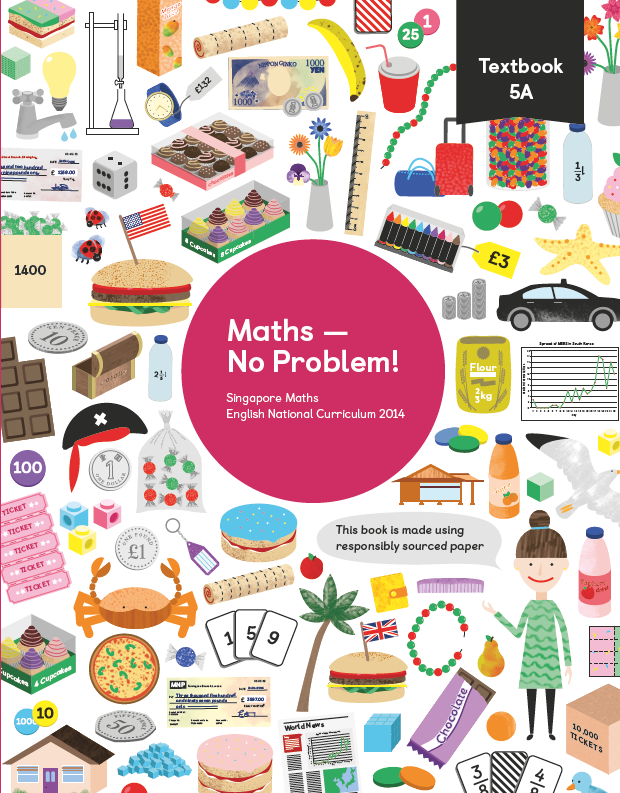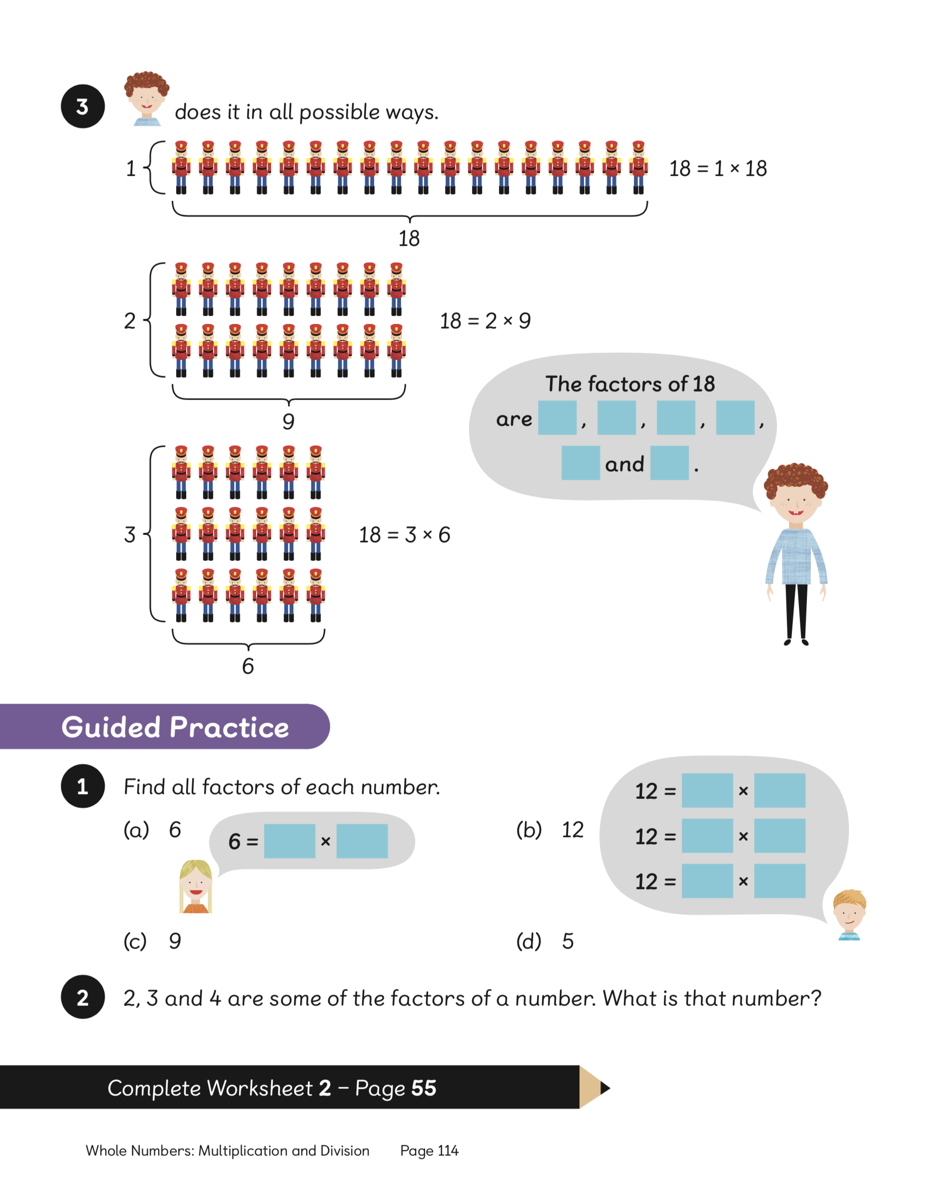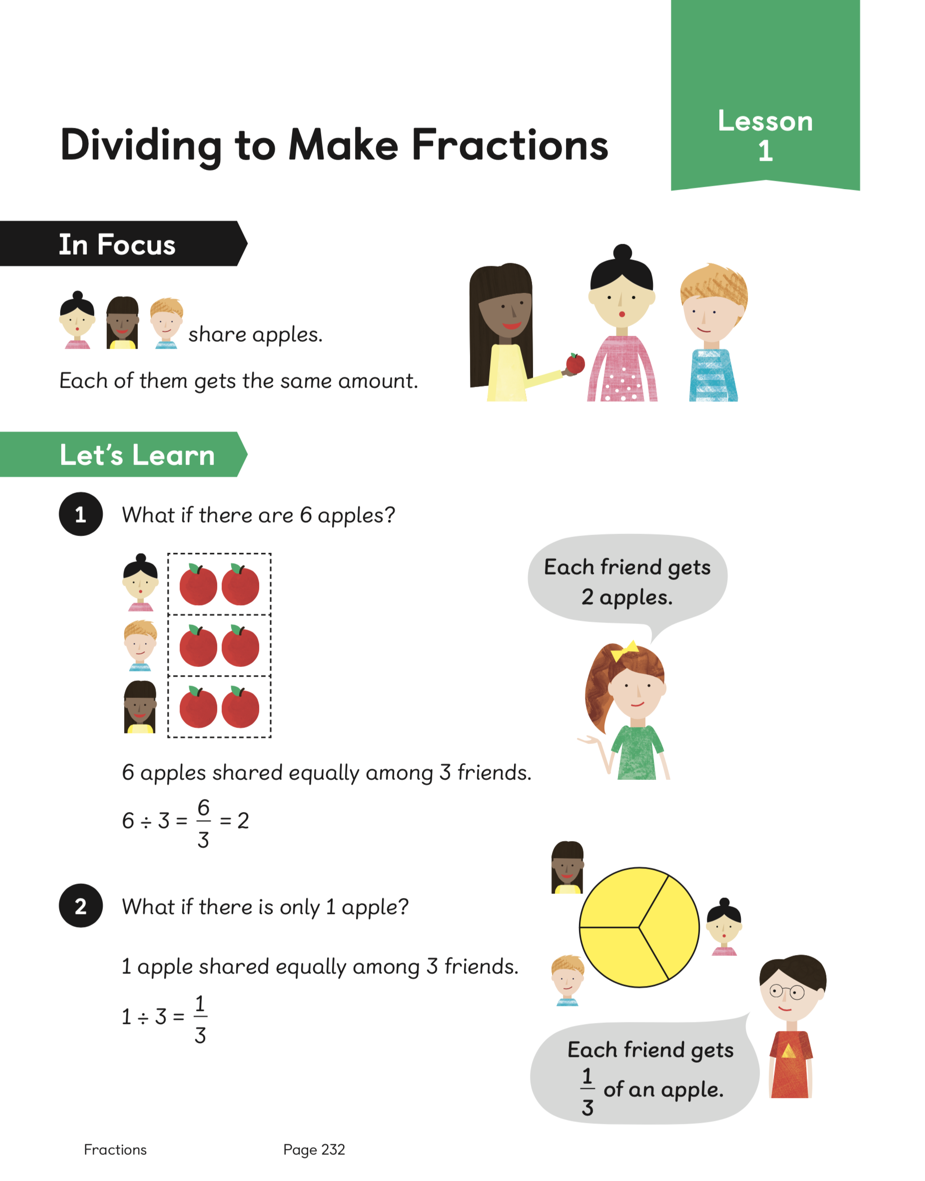Textbook 5A# Maths — No Problem!Textbook 5A

## £9.99

ISBN: 9781910504147
Pages: 284
Availability:
In stock
Qty:

• Lesson 1 - Reading and Writing Numbers to 100 000

To be able to read and represent numbers to 100 000.

• Lesson 2 - Reading and Writing Numbers to 1 000 000

To be able to read and represent numbers to 1 000 000.

• Lesson 3 - Reading and Writing Numbers to 1 000 000

To be able to read and represent numbers to 1 000 000 using number discs.

• Lesson 4 - Comparing Numbers to 1 000 000

To be able to compare numbers to 1 000 000 using place value.

• Lesson 5 - Comparing Numbers to 1 000 000

To be able to compare numbers to 1 000 000 using place value.

• Lesson 6 - Comparing Numbers to 1 000 000

To be able to compare numbers to 1 000 000 using pictorial representations and proportionality.

• Lesson 7 - Comparing Numbers to 1 000 000

To be able to compare numbers to 1 000 000 from pictorial representations, using lists and number lines.

• Lesson 8 - Making Number Patterns

To be able to make and identify patterns in numbers using knowledge of place value.

• Lesson 9 - Making Number Patterns

To be able to make number patterns that decrease in multiples of 10 000 or 100 000.

• Lesson 10 - Rounding Numbers

To be able to round numbers to the nearest 10 000 using number lines and bar graphs.

• Lesson 11 - Rounding Numbers

To be able to round numbers to the nearest 100 000 using number lines and bar graphs.

• Lesson 12 - Rounding Numbers

To be able to round numbers to the nearest 100, 1000, 10 000 and 100 000 using number lines.

• Lesson 13 - Chapter Consolidation

To be able to apply knowledge of numbers to 1 000 000 to solve problems.

## Author(s)

Consultant and Author: Dr. Yeap Ban Har
UK Consultant: Dr. Anne Hermanson